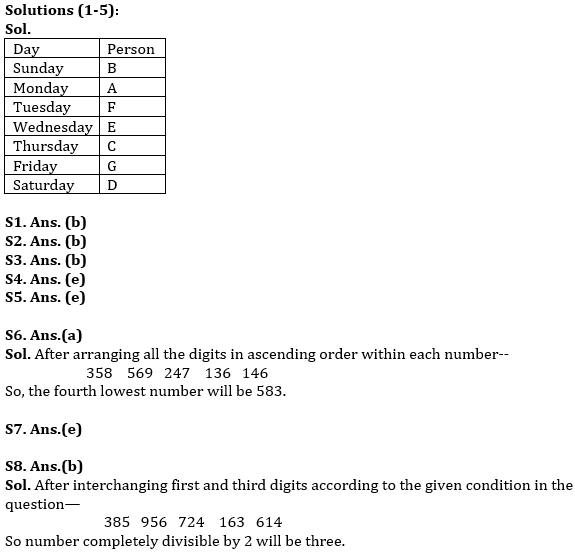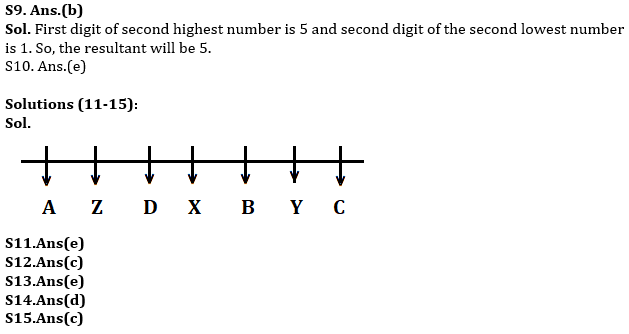Latest Banking jobs   »

# Reasoning Ability Quiz For RBI Assistant Prelims 2022- 25th March

Directions (1-5): Study the information carefully and answer the following questions.
Seven persons A, B, C, D, E, F, G have weekly off on different days of the week starting from Sunday. F has off on one of the days after B. A does not has off on first day of the week. Not more than three persons have off after E. G has off immediate before D and after C. C doesn’t have off before E. More than 3 persons have off between A and D.

Q1. Who among the following has off on Friday?
(a) A
(b) G
(c) C
(d) D
(e) None of these

Q2. How many persons have off before F?
(a) 1
(b) 2
(c) 3
(d) 4
(e) none

Q3. How many persons have off between E and the one who has off immediate before A?
(a) 1
(b) 2
(c) 3
(d) 4
(e) none

Q4. Who among the following has off immediate after D?
(a) A
(b) B
(c) C
(d) G
(e) None

Q5. Which of the statement is not true regarding D?
(a) A doesn’t has off after D.
(b) B has off before D.
(c) Only one person has off between C and D.
(d) D has off immediate after G.
(e) All are true

Directions (6-10): The following questions are based on the five three digits numbers given below:

583   659   427   361   416

Q6. If in each number, all the three digits are arranged in ascending order within the number, which of the following will be the fourth lowest number?
(a) 583
(b) 659
(c) 427
(d) 361
(e) 416

Q7. If first digit will be changed (replace) with third digit, then second with first digit and after that third digit with the second digit in each number, then which number will be the lowest?
(a) 583
(b) 659
(c) 427
(d) 361
(e) 416

Q8. If the position of first and third digits of the numbers are interchanged with each number, then how many numbers thus formed will be completely divisible by 2?
(a) None
(b) Three
(c) More than three
(d) One
(e) Two

Q9. What will be result if first digit of second highest number is divided by second digit of second lowest number?
(a) 4
(b) 5
(c) 7
(d) 2
(e) 1

Q10. If in each number, both first and second digits are added and then third digit will be subtracted from that addition then which of the following will be the highest resultant?
(a) 4
(b) 2
(c) 1
(d) 8
(e) 10

Directions (11-15): Study the information carefully and answer the questions given below.
Seven persons are sitting in a linear row. All of them are facing south. Equal number of persons sits to left and right of X. Two persons sit between X and C. D sits second to the right of B. More than three persons sit between A and Y, who doesn’t sit at extreme end of row. Z is not immediate neighbor of B.

Q11. Who among the following person sits fourth to the right of Z?
(a) A
(b) X
(c) B
(d)D
(e) None of these

Q12. Who among the following is immediate left of D?
(a) Z
(b) B
(c) X
(d) A
(e) None of these

Q13. How many persons sit between Y and B?
(a) Three
(b) Four
(c) one
(d) Two
(e) None

Q14. Four of the following five are alike in certain way based from a group, find the one that does not belong to that group?
(a) X-Z
(b) B-D
(c) Y-X
(d)A-Z
(e) B-C

Q15.If A and D change their places then which of the following person sit immediate right of A?
(a) Y
(b) B
(c) Z
(d) C
(e) None of these

Solutions